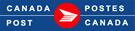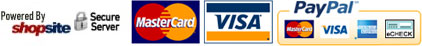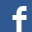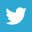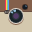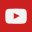25oz / 739ml
Can\$19.99
25oz / 739ml
Can\$19.99
25oz / 739ml
Can\$19.99
Each
Can\$6.99
Each
Can\$6.99
Each
Can\$6.99
Each
Can\$9.99
Each
Can\$9.99
Each
Can\$9.99
Each
Can\$9.99
Each
Can\$9.99
Each
Can\$9.99
Each
Can\$12.99
Each
Can\$12.99
Each
Can\$2.99
Each
Can\$2.99
Each
Can\$2.99
Each
Can\$1.99
Each
Can\$12.99
Each
Can\$12.99
Each
Can\$12.99
Each
Can\$12.99
Each
Can\$12.99
20 oz
Can\$2.99
20 oz
Can\$2.99
20 oz
Can\$2.99
22 oz
Can\$2.99
22 oz
Can\$2.99
Each
Can\$16.99
Each
Can\$24.99
Each
Can\$24.99
Each
Can\$24.99
Each
Can\$24.99
Each
Can\$24.99
Each
Can\$29.99
Each
Can\$24.99
Each
Can\$29.99
Each
Can\$24.99
Each
Can\$24.99
Each
Can\$24.99
Each
Can\$24.99
Large
Can\$24.99
Each
Can\$24.99
Each
Can\$29.99
Each
Can\$19.99
Each
Can\$19.99
Each
Can\$19.99
Each
Can\$39.99
Each
Can\$79.99
Each
Can\$79.99
Each
Can\$29.99
Each
Can\$12.99
Each
Can\$12.99
Each
Can\$12.99
Each
Can\$12.99
Each
Can\$12.99
Each
Can\$12.99
Each
Can\$12.99
Each
Can\$12.99
Each
Can\$12.99
Each
Can\$12.99
Each
Can\$9.99
Each
Can\$9.99
Each
Can\$39.99
Each
Can\$39.99
Each
Can\$39.99
Each
Can\$29.99
Each
Can\$29.99
Each
Can\$29.99
Each
Can\$29.99
Each
Can\$9.99
Each
Can\$4.99
Each
Can\$12.99
Each
Can\$12.99
Each
Can\$12.99
Each
Can\$12.99
Each
Can\$12.99
Each
Can\$19.99
Each
Can\$19.99
Each
Can\$19.99
Each
Can\$29.99
Each
Can\$29.99
Each
Can\$16.99
Each
Can\$16.99
Each
Can\$16.99
Each
Can\$16.99
Each
Can\$16.99
Each
Can\$16.99
Each
Can\$16.99
Each
Can\$16.99
Each
Can\$16.99
Each
Can\$19.99
Each
Can\$19.99
Each
Can\$15.99
Each
Can\$15.99
Each
Can\$9.99
Each
Can\$15.99
Each
Can\$4.99
Each
Can\$4.99
Each
Can\$12.99
Each
Can\$4.99
2 x 1 Block
Can\$9.99
Pair
Can\$7.99
Pair
Can\$12.99
Pair
Can\$12.99
Pair
Can\$12.99
Pair
Can\$19.99
Pair
Can\$24.99
Pair
Can\$14.99
Each
Can\$14.99
Each
Can\$9.99
Each
Can\$9.99
Each
Can\$9.99
Each
Can\$8.99
Each
Can\$3.99
Each
Can\$4.99
Each
Can\$4.99
Each
Can\$4.99
Each
Can\$4.99
Each
Can\$39.99
Each
Can\$9.99
Each
Can\$12.99